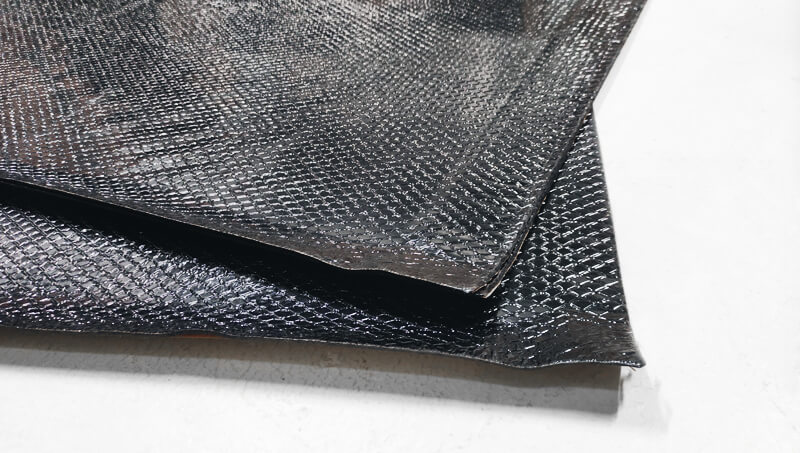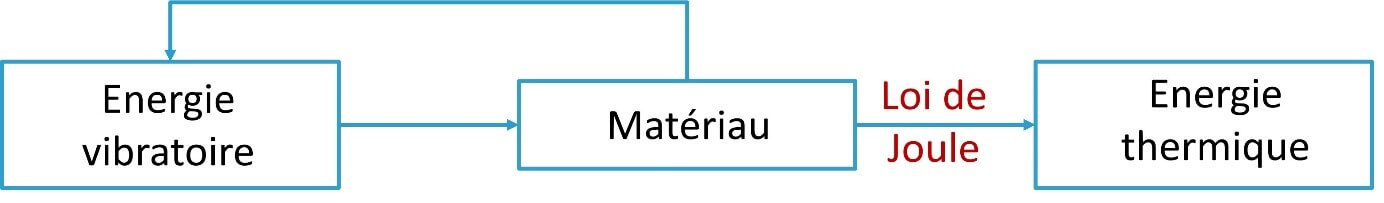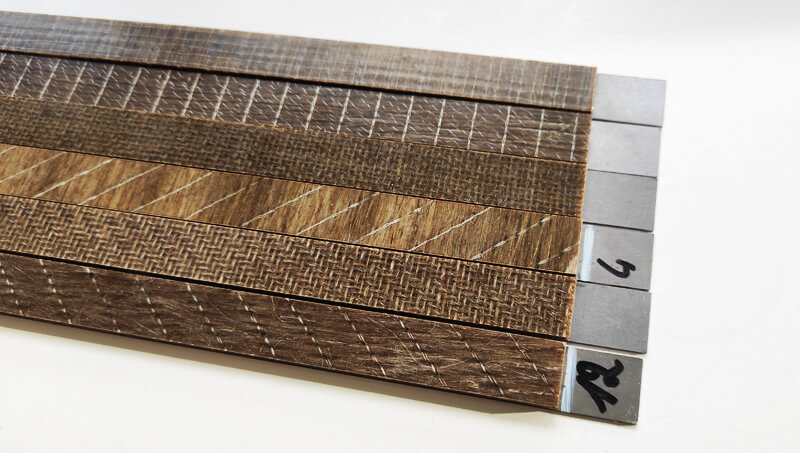Select Page

# Acoustic and vibration dampingThe CEVAA improves the damping of the materials. It measures, in fact, the loss factor and the modulus of elasticity of storage.

## Damping

### Damping principles

In theory, without a source of internal or external damping, a structure which would vibrate on one of its resonant frequencies, would have an infinite amplitude response.

In reality, a vibrating structure has a multitude of sources of damping of its vibrations, such as:

• Friction within the microstructure of the materials constituting it,
• Air or water friction if the structure is submerged
• Friction at the interfaces between parts, in particular at the level of the connections (screwed, welded, glued, etc.).

Different damping models exist and make it possible to quantify the power of dissipation of vibrational energy. The hysteretic damping model, also called structural damping, is mainly used to quantify the damping of materials, through the loss factor. The viscous damping model is used to quantify the damping observed during experimental measurements via a logarithmic decrement type approach or a modal analysis.

#### Joule’s Law

Whatever the model, the higher the damping, the lower the amplitude of the vibrations. From an energy point of view, the dissipation of vibrations is explained by the transformation of the mechanical energy transmitted to the material into thermal energy within its microstructure. This is Joule’s law. The loss factor represents the part of energy transformed at each period of the vibration of the mechanical waves.## Intrinsic damping of materials

A few orders of magnitude of the materials intrinsic damping, i.e. loss factor:

• Steel = 2/1000
• Aluminum = 1/10000
• Plastic between 1 and 10%
• Cork = 15%
• Rubber between 5 and 30%
• Viscoelastic > 10%

The higher the loss factor, the more its value depends on environmental factors (frequency, temperature, humidity) and preload. The orders of magnitude given above should be used with caution and simply make it possible to observe an initial classification of materials with respect to their vibration dissipation power.## Determine Material Loss Factor

To precisely determine the loss factor of materials, the CEVAA has two complementary test means:

• Oberst method for measuring the composite loss factor by an indirect method of the resonant type, according to the ISO 6721-3 standard
• Viscoanalyzer (DMA) allowing the measurement of the loss factor and the modulus of elasticity of storage by a direct method, out of resonance, according to the standards ISO 6721-4, ISO 6721-5 and ISO 6721-6.

Each method has advantages and disadvantages. The CEVAA complements the Oberst method with RKU theory to extract from measured data the intrinsic loss factor and the storage modulus of elasticity of the tested sample.

## MATERIALS CHARACTERIZATION BENCHES

Poisson's ratio characterization bench

Dynamic mechanical analysis (DMA)